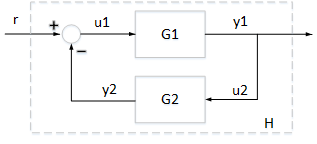## Feedback Interconnection of Passive Systems

This example illustrates the properties of a feedback interconnection of passive systems.

### Feedback Interconnection of Passive Systems

Consider an interconnection of two subsystems ${G}_{1}$ and ${G}_{2}$ in feedback. The interconnected system $H$ maps the input $r$ to the output ${y}_{1}$.If both systems ${G}_{1}$ and ${G}_{2}$ are passive, then the interconnected system $H$ is guaranteed to be passive. Take for example

`${G}_{1}\left(s\right)=\frac{{s}^{2}+s+1}{{s}^{2}+s+4},\phantom{\rule{0.2777777777777778em}{0ex}}\phantom{\rule{0.2777777777777778em}{0ex}}\phantom{\rule{0.2777777777777778em}{0ex}}{G}_{2}\left(s\right)=\frac{s+2}{s+5}.$`

Both systems are passive as confirmed by

```G1 = tf([1,1,1],[1,1,4]); isPassive(G1)```
```ans = logical 1 ```
```G2 = tf([1,2],[1,5]); isPassive(G2)```
```ans = logical 1 ```

The interconnected system is therefore passive.

```H = feedback(G1,G2); isPassive(H)```
```ans = logical 1 ```

This is confirmed by verifying that the Nyquist plot of $H$ is positive real.

`nyquist(H)`### Passivity Indices for Feedback Interconnection

There is a relationship between the passivity indices of ${G}_{1}$ and ${G}_{2}$ and the passivity indices of the interconnected system $H$. Let ${\nu }_{1}$ and ${\nu }_{2}$ denote the input passivity indices for ${G}_{1}$ and ${G}_{2}$, and let ${\rho }_{1}$ and ${\rho }_{2}$ denote the output passivity indices. If all these indices are positive, then the input passivity index $\nu$ and the output passivity index $\rho$ for the feedback interconnection $H$ satisfy

`$\nu \ge \frac{{\nu }_{1}{\rho }_{2}}{{\nu }_{1}+{\rho }_{2}},\phantom{\rule{0.2777777777777778em}{0ex}}\phantom{\rule{0.2777777777777778em}{0ex}}\phantom{\rule{0.2777777777777778em}{0ex}}\rho \ge {\rho }_{1}+{\nu }_{2}.$`

In other words, we can infer some minimum level of input and output passivity for the closed-loop system $H$ from the input and output passivity indices of ${G}_{1}$ and ${G}_{2}$. For details, see the paper by Zhu, F. and Xia, M and Antsaklis, P.J., "Passivity analysis and passivation of feedback systems using passivity indices," American Control Conference , 2014, pp. 1833-1838. Verify the lower bound for the input passivity index $\nu$.

```% Input passivity index for G1 nu1 = getPassiveIndex(G1,'input'); % Output passivity index for G2 rho2 = getPassiveIndex(G2,'output'); % Input passivity index for H nu = getPassiveIndex(H,'input')```
```nu = 0.1293 ```
```% Lower bound nu1*rho2/(nu1+rho2)```
```ans = 7.1402e-11 ```

Similarly, verify the lower bound for the output passivity index of $H$.

```% Output passivity index for G1 rho1 = getPassiveIndex(G1,'output'); % Input passivity index for G2 nu2 = getPassiveIndex(G2,'input'); % Output passivity index for H rho = getPassiveIndex(H,'output')```
```rho = 0.4441 ```
```% Lower bound rho1+nu2```
```ans = 0.4000 ```